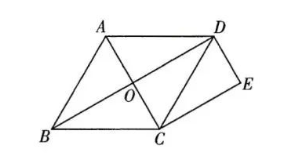【答案】 证明: $\because$ 菱形 $A B C D$ 的对角线 $A C$ 和 $B D$ 相交于点 $O$,
\begin{aligned} & \therefore O C=\frac{1}{2} A C, A C \perp B D . \\ & \because D E=\frac{1}{2} A C, \therefore D E=O C . \\ & \because D E / / A C, \therefore \text { 四边形 } O C E D \text { 是平行四边形. } \\ & \because A C \perp B D \text { 于点 } O, \therefore \angle C O D=90^{\circ} . \\ & \therefore \text { 四边形 } O C E D \text { 是矩形. } \end{aligned}